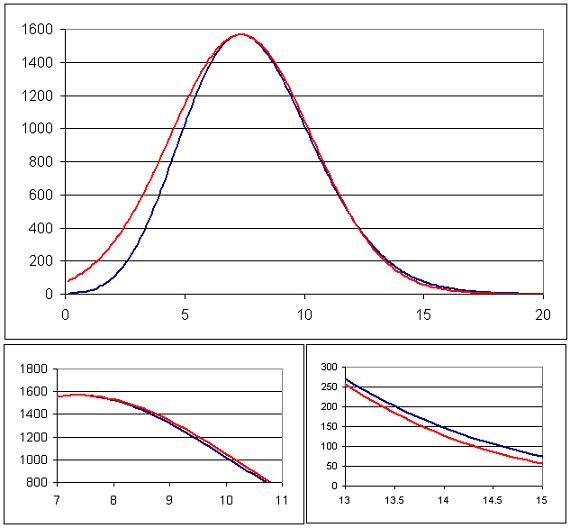#### You may also like### How Many Solutions?

Find all the solutions to the this equation.### Quartics

Investigate the graphs of y = [1 + (x - t)^2][1 + (x + t^)2] as the parameter t varies.### Power Up

Show without recourse to any calculating aid that 7^{1/2} + 7^{1/3} + 7^{1/4} < 7 and 4^{1/2} + 4^{1/3} + 4^{1/4} > 4 . Sketch the graph of f(x) = x^{1/2} + x^{1/3} + x^{1/4} -x

# A Close Match

##### Age 16 to 18Challenge Level

I was delighted to receive this solution from Niharika Paul, one of our younger solvers.

The actual functions plotted were as follows:

Two functions $f(x)$ and $g(x)$ were plotted on the same axes, where
$$f(x) =\left(\frac{20}{x}\right)^x\quad \quad g(x) = 1568\exp\left(-\frac{(x-7.3576)^2}{17.6232}\right)$$
The coefficients in $g(x)$ were chosen so as to make the function $g(x)$ match $f(x)$ as closely as possible for points past the maximum of $f(x)$

Their charts at various points are